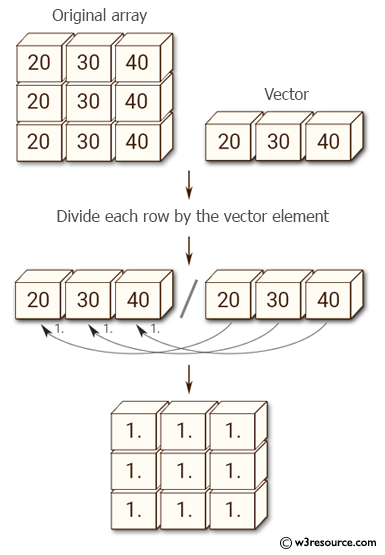﻿ NumPy: Divide each row by a vector element - w3resource# NumPy: Divide each row by a vector element

## NumPy: Array Object Exercise-96 with Solution

Write a NumPy program to divide each row by a vector element.

Pictorial Presentation:Sample Solution:

Python Code:

``````import numpy as np
x = np.array([[20,20,20],[30,30,30],[40,40,40]])
print("Original array:")
print(x)
v = np.array([20,30,40])
print("Vector:")
print(v)
print(x / v[:,None])
```
```

Sample Output:

```Original array:
[[20 20 20]
[30 30 30]
[40 40 40]]
Vector:
[20 30 40]
[[ 1.  1.  1.]
[ 1.  1.  1.]
[ 1.  1.  1.]]
```

Python Code Editor:

Have another way to solve this solution? Contribute your code (and comments) through Disqus.

What is the difficulty level of this exercise?

Test your Python skills with w3resource's quiz

﻿

## Python: Tips of the Day

List comprehension:

```>>> m = [x ** 2 for x in range(5)]
>>> m
[0, 1, 4, 9, 16]
```